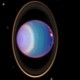Connect with us

# This 6 Digit Number is a Key to the UniversePublished

onI find it fascinating that for some reason, 22 divided by 7 = roughly Pi, or 3.142857.

Pi is of course a number that is arguably the most important and mysterious number in creation, being the number of the circle or sphere. Modestly summarized by Wikipedia:

“The number π (/ˈp/) is a mathematical constant. Originally defined as the ratio of a circle’s circumference to its diameter, it now has various equivalent definitions and appears in many formulas in all areas of mathematics and physics. It is approximately equal to 3.14159. It has been represented by the Greek letter “π” since the mid-18th century, though it is also sometimes spelled out as “pi“.”

It was allegedly proved by mathematicians as far back as the 3rd Century BC that 22 divided by 7 is a very slight overestimation of Pi. That equation equals 3.142857 (continuing onto more digits), and Pi is actually 3.141592, continuing on.But when I researched the following 6 digits after 3 in 22 divided by 7, the number 142,857, I came across some very interesting facts.

1/7th equals 0.142857142857142857…

So one seventh of anything is this number, .142857.

Even stranger, if you multiply 142,857 by 7, for some reason you get 999,999.

If you add 142 + 857, you get 999.`

And if you multiply this number by any number 1 – 6, the same digits repeat themselves in the result. For example:

142857 * 2 = 285714
142857 * 3 = 428571
142857 * 4 = 571428
142857 * 5 = 714285
142857 * 6 = 857142

Any number that isn’t evenly divisible by 7 divided by 7, ends up bearing a number with infinitely repeating decimals of 142857: 142857 repeating endlessly. It is a Cyclic Number, one of the best known.

Try it out: go to the calculator on your phone or computer and try these equations. It’s like peering into the coding of our universe.

In space, objects are rounded into spheres by gravity and other forces. The sphere or circle is a symbol of life, and its number is Pi, roughly 22 divided by 7.

This number 142,857 is encoded into the very shape of a sphere or a circle, and its relation to the number 7 makes me wonder if this isn’t a reason the number 7 is so revered throughout history.

This number says something very strange about the nature of our reality. Does it not suggest that our world is somehow coded?

For more interesting info on Pi, Marty Leeds is a fascinating researcher with a YouTube channel full of info on numbers, the occult, and all kinds of other things.

(Image credit: CIG, One Million Digits of Pi)